# sklearn.svm.SVC¶

class sklearn.svm.SVC(C=1.0, kernel='rbf', degree=3, gamma='auto_deprecated', coef0=0.0, shrinking=True, probability=False, tol=0.001, cache_size=200, class_weight=None, verbose=False, max_iter=-1, decision_function_shape='ovr', random_state=None)[source]

C-Support Vector Classification.

The implementation is based on libsvm. The fit time complexity is more than quadratic with the number of samples which makes it hard to scale to dataset with more than a couple of 10000 samples.

The multiclass support is handled according to a one-vs-one scheme.

For details on the precise mathematical formulation of the provided kernel functions and how gamma, coef0 and degree affect each other, see the corresponding section in the narrative documentation: Kernel functions.

Read more in the User Guide.

Parameters: C : float, optional (default=1.0) Penalty parameter C of the error term. kernel : string, optional (default=’rbf’) Specifies the kernel type to be used in the algorithm. It must be one of ‘linear’, ‘poly’, ‘rbf’, ‘sigmoid’, ‘precomputed’ or a callable. If none is given, ‘rbf’ will be used. If a callable is given it is used to pre-compute the kernel matrix from data matrices; that matrix should be an array of shape (n_samples, n_samples). degree : int, optional (default=3) Degree of the polynomial kernel function (‘poly’). Ignored by all other kernels. gamma : float, optional (default=’auto’) Kernel coefficient for ‘rbf’, ‘poly’ and ‘sigmoid’. Current default is ‘auto’ which uses 1 / n_features, if gamma='scale' is passed then it uses 1 / (n_features * X.var()) as value of gamma. The current default of gamma, ‘auto’, will change to ‘scale’ in version 0.22. ‘auto_deprecated’, a deprecated version of ‘auto’ is used as a default indicating that no explicit value of gamma was passed. coef0 : float, optional (default=0.0) Independent term in kernel function. It is only significant in ‘poly’ and ‘sigmoid’. shrinking : boolean, optional (default=True) Whether to use the shrinking heuristic. probability : boolean, optional (default=False) Whether to enable probability estimates. This must be enabled prior to calling fit, and will slow down that method. tol : float, optional (default=1e-3) Tolerance for stopping criterion. cache_size : float, optional Specify the size of the kernel cache (in MB). class_weight : {dict, ‘balanced’}, optional Set the parameter C of class i to class_weight[i]*C for SVC. If not given, all classes are supposed to have weight one. The “balanced” mode uses the values of y to automatically adjust weights inversely proportional to class frequencies in the input data as n_samples / (n_classes * np.bincount(y)) verbose : bool, default: False Enable verbose output. Note that this setting takes advantage of a per-process runtime setting in libsvm that, if enabled, may not work properly in a multithreaded context. max_iter : int, optional (default=-1) Hard limit on iterations within solver, or -1 for no limit. decision_function_shape : ‘ovo’, ‘ovr’, default=’ovr’ Whether to return a one-vs-rest (‘ovr’) decision function of shape (n_samples, n_classes) as all other classifiers, or the original one-vs-one (‘ovo’) decision function of libsvm which has shape (n_samples, n_classes * (n_classes - 1) / 2). However, one-vs-one (‘ovo’) is always used as multi-class strategy. Changed in version 0.19: decision_function_shape is ‘ovr’ by default. New in version 0.17: decision_function_shape=’ovr’ is recommended. Changed in version 0.17: Deprecated decision_function_shape=’ovo’ and None. random_state : int, RandomState instance or None, optional (default=None) The seed of the pseudo random number generator used when shuffling the data for probability estimates. If int, random_state is the seed used by the random number generator; If RandomState instance, random_state is the random number generator; If None, the random number generator is the RandomState instance used by np.random. support_ : array-like, shape = [n_SV] Indices of support vectors. support_vectors_ : array-like, shape = [n_SV, n_features] Support vectors. n_support_ : array-like, dtype=int32, shape = [n_class] Number of support vectors for each class. dual_coef_ : array, shape = [n_class-1, n_SV] Coefficients of the support vector in the decision function. For multiclass, coefficient for all 1-vs-1 classifiers. The layout of the coefficients in the multiclass case is somewhat non-trivial. See the section about multi-class classification in the SVM section of the User Guide for details. coef_ : array, shape = [n_class * (n_class-1) / 2, n_features] Weights assigned to the features (coefficients in the primal problem). This is only available in the case of a linear kernel. coef_ is a readonly property derived from dual_coef_ and support_vectors_. intercept_ : array, shape = [n_class * (n_class-1) / 2] Constants in decision function. fit_status_ : int 0 if correctly fitted, 1 otherwise (will raise warning) probA_ : array, shape = [n_class * (n_class-1) / 2] probB_ : array, shape = [n_class * (n_class-1) / 2] If probability=True, the parameters learned in Platt scaling to produce probability estimates from decision values. If probability=False, an empty array. Platt scaling uses the logistic function 1 / (1 + exp(decision_value * probA_ + probB_)) where probA_ and probB_ are learned from the dataset . For more information on the multiclass case and training procedure see section 8 of .

See also

SVR
Support Vector Machine for Regression implemented using libsvm.
LinearSVC
Scalable Linear Support Vector Machine for classification implemented using liblinear. Check the See also section of LinearSVC for more comparison element.

References

Examples

>>> import numpy as np
>>> X = np.array([[-1, -1], [-2, -1], [1, 1], [2, 1]])
>>> y = np.array([1, 1, 2, 2])
>>> from sklearn.svm import SVC
>>> clf = SVC(gamma='auto')
>>> clf.fit(X, y)
SVC(C=1.0, cache_size=200, class_weight=None, coef0=0.0,
decision_function_shape='ovr', degree=3, gamma='auto', kernel='rbf',
max_iter=-1, probability=False, random_state=None, shrinking=True,
tol=0.001, verbose=False)
>>> print(clf.predict([[-0.8, -1]]))



Methods

 decision_function(X) Evaluates the decision function for the samples in X. fit(X, y[, sample_weight]) Fit the SVM model according to the given training data. get_params([deep]) Get parameters for this estimator. predict(X) Perform classification on samples in X. score(X, y[, sample_weight]) Returns the mean accuracy on the given test data and labels. set_params(**params) Set the parameters of this estimator.
__init__(C=1.0, kernel='rbf', degree=3, gamma='auto_deprecated', coef0=0.0, shrinking=True, probability=False, tol=0.001, cache_size=200, class_weight=None, verbose=False, max_iter=-1, decision_function_shape='ovr', random_state=None)[source]
decision_function(X)[source]

Evaluates the decision function for the samples in X.

Parameters: X : array-like, shape (n_samples, n_features) X : array-like, shape (n_samples, n_classes * (n_classes-1) / 2) Returns the decision function of the sample for each class in the model. If decision_function_shape=’ovr’, the shape is (n_samples, n_classes).

Notes

If decision_function_shape=’ovo’, the function values are proportional to the distance of the samples X to the separating hyperplane. If the exact distances are required, divide the function values by the norm of the weight vector (coef_). See also this question for further details.

fit(X, y, sample_weight=None)[source]

Fit the SVM model according to the given training data.

Parameters: X : {array-like, sparse matrix}, shape (n_samples, n_features) Training vectors, where n_samples is the number of samples and n_features is the number of features. For kernel=”precomputed”, the expected shape of X is (n_samples, n_samples). y : array-like, shape (n_samples,) Target values (class labels in classification, real numbers in regression) sample_weight : array-like, shape (n_samples,) Per-sample weights. Rescale C per sample. Higher weights force the classifier to put more emphasis on these points. self : object

Notes

If X and y are not C-ordered and contiguous arrays of np.float64 and X is not a scipy.sparse.csr_matrix, X and/or y may be copied.

If X is a dense array, then the other methods will not support sparse matrices as input.

get_params(deep=True)[source]

Get parameters for this estimator.

Parameters: deep : boolean, optional If True, will return the parameters for this estimator and contained subobjects that are estimators. params : mapping of string to any Parameter names mapped to their values.
predict(X)[source]

Perform classification on samples in X.

For an one-class model, +1 or -1 is returned.

Parameters: X : {array-like, sparse matrix}, shape (n_samples, n_features) For kernel=”precomputed”, the expected shape of X is [n_samples_test, n_samples_train] y_pred : array, shape (n_samples,) Class labels for samples in X.
predict_log_proba

Compute log probabilities of possible outcomes for samples in X.

The model need to have probability information computed at training time: fit with attribute probability set to True.

Parameters: X : array-like, shape (n_samples, n_features) For kernel=”precomputed”, the expected shape of X is [n_samples_test, n_samples_train] T : array-like, shape (n_samples, n_classes) Returns the log-probabilities of the sample for each class in the model. The columns correspond to the classes in sorted order, as they appear in the attribute classes_.

Notes

The probability model is created using cross validation, so the results can be slightly different than those obtained by predict. Also, it will produce meaningless results on very small datasets.

predict_proba

Compute probabilities of possible outcomes for samples in X.

The model need to have probability information computed at training time: fit with attribute probability set to True.

Parameters: X : array-like, shape (n_samples, n_features) For kernel=”precomputed”, the expected shape of X is [n_samples_test, n_samples_train] T : array-like, shape (n_samples, n_classes) Returns the probability of the sample for each class in the model. The columns correspond to the classes in sorted order, as they appear in the attribute classes_.

Notes

The probability model is created using cross validation, so the results can be slightly different than those obtained by predict. Also, it will produce meaningless results on very small datasets.

score(X, y, sample_weight=None)[source]

Returns the mean accuracy on the given test data and labels.

In multi-label classification, this is the subset accuracy which is a harsh metric since you require for each sample that each label set be correctly predicted.

Parameters: X : array-like, shape = (n_samples, n_features) Test samples. y : array-like, shape = (n_samples) or (n_samples, n_outputs) True labels for X. sample_weight : array-like, shape = [n_samples], optional Sample weights. score : float Mean accuracy of self.predict(X) wrt. y.
set_params(**params)[source]

Set the parameters of this estimator.

The method works on simple estimators as well as on nested objects (such as pipelines). The latter have parameters of the form <component>__<parameter> so that it’s possible to update each component of a nested object.

Returns: self

## Examples using sklearn.svm.SVC¶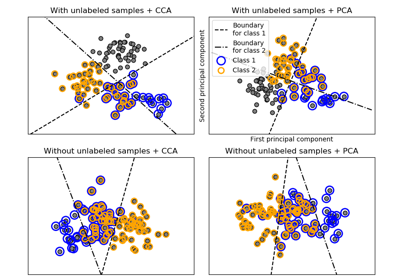Multilabel classification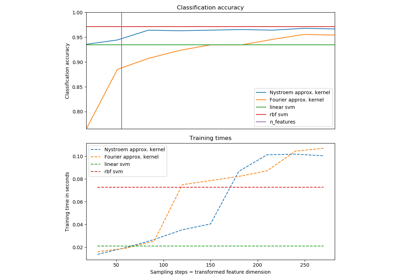Explicit feature map approximation for RBF kernels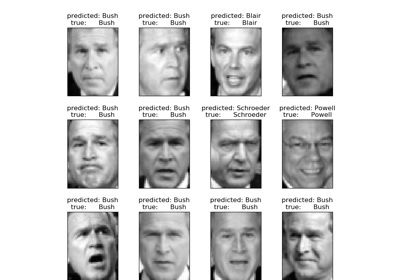Faces recognition example using eigenfaces and SVMsLibsvm GUI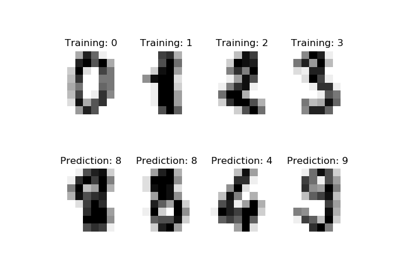Recognizing hand-written digits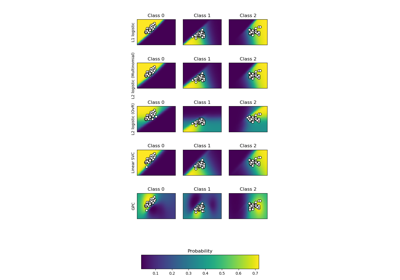Plot classification probability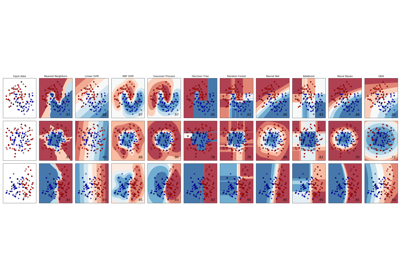Classifier comparisonConcatenating multiple feature extraction methods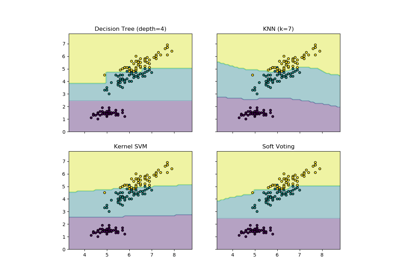Plot the decision boundaries of a VotingClassifier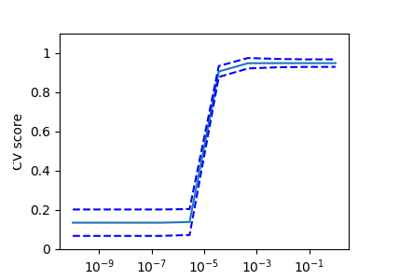Cross-validation on Digits Dataset Exercise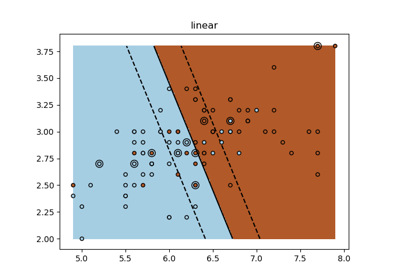SVM Exercise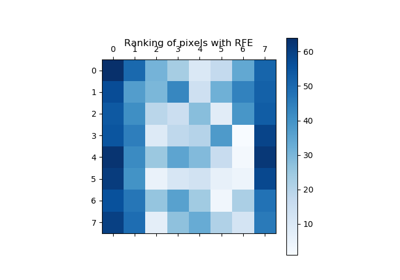Recursive feature eliminationPipeline Anova SVM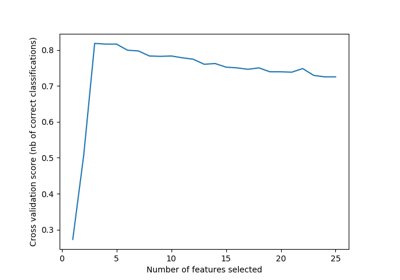Recursive feature elimination with cross-validation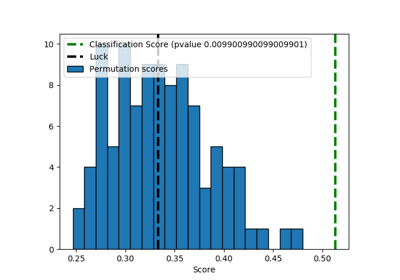Test with permutations the significance of a classification score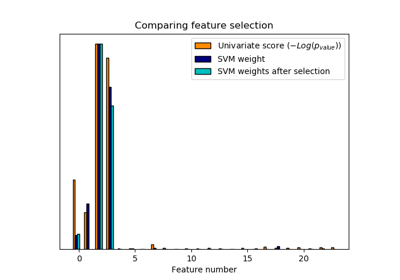Univariate Feature Selection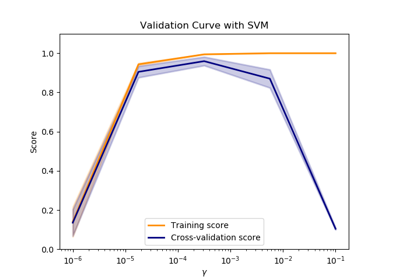Plotting Validation CurvesParameter estimation using grid search with cross-validation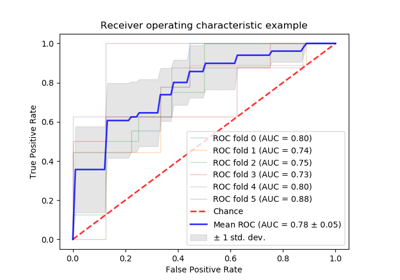Receiver Operating Characteristic (ROC) with cross validation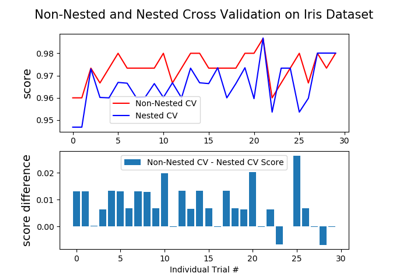Nested versus non-nested cross-validation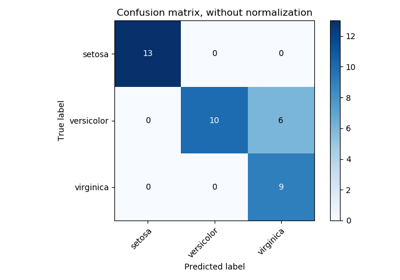Confusion matrix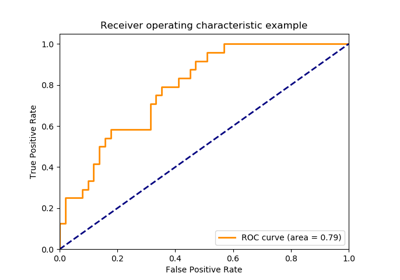Receiver Operating Characteristic (ROC)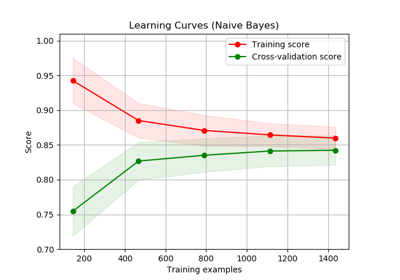Plotting Learning Curves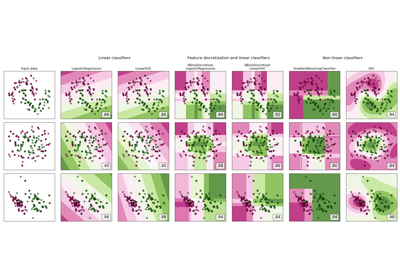Feature discretization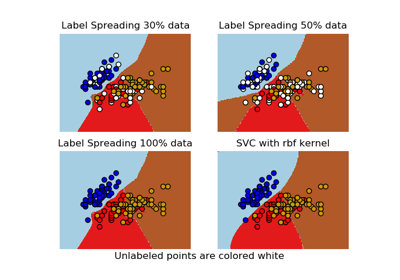Decision boundary of label propagation versus SVM on the Iris dataset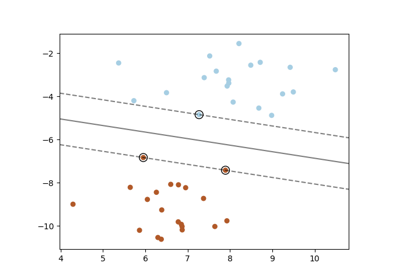SVM: Maximum margin separating hyperplane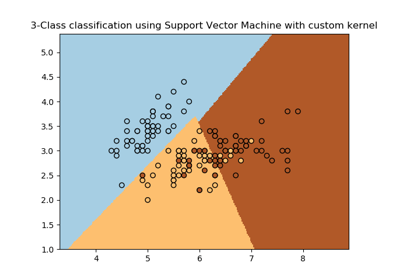SVM with custom kernel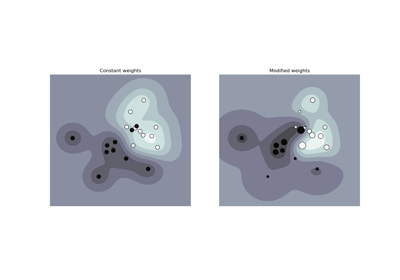SVM: Weighted samples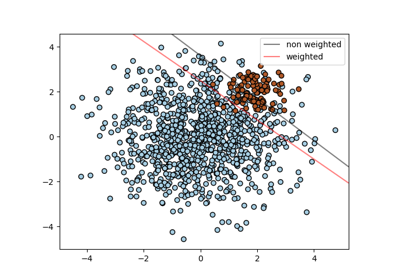SVM: Separating hyperplane for unbalanced classes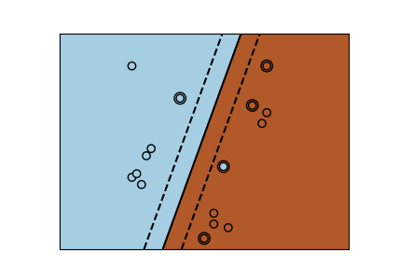SVM-Kernels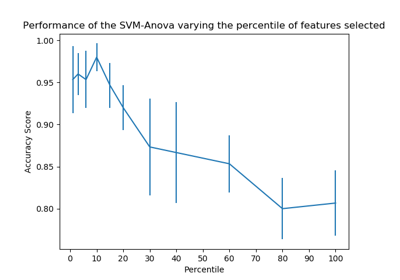SVM-Anova: SVM with univariate feature selection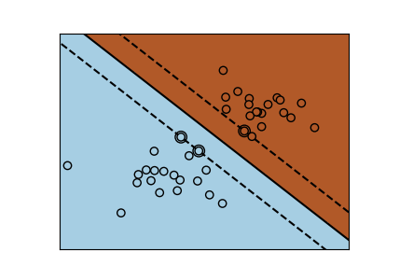SVM Margins Example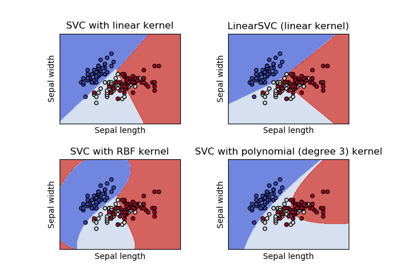Plot different SVM classifiers in the iris dataset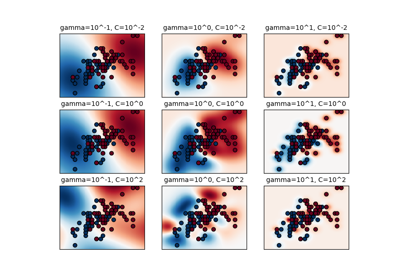RBF SVM parameters